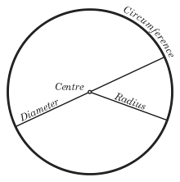## FANDOM

120 PagesAn illustration of a circle showing the circumference, centre, diameter and radius. Circles are completely round shapes where every point from the center of the circle to its circumference is the same distance.

## Relationship with pi

Pi can be used to calculate the circumference of a circle, which is similar to the perimeter of other shapes. The circumference of a circle is always exactly pi (3.1415...) times the diameter of the circle, no matter how large or the small the circle is. For example, a circle with diameter 10 would have an area of 31.415...

Community content is available under CC-BY-SA unless otherwise noted.